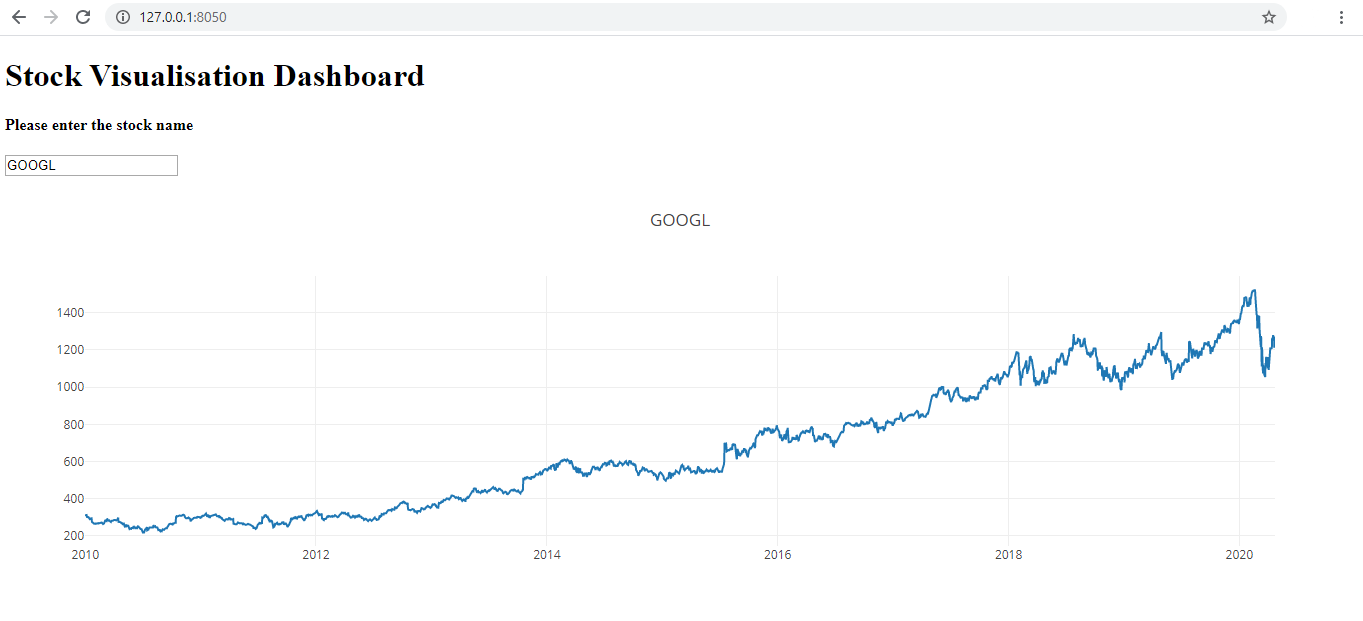# Python – Stock Data Visualisation

• Difficulty Level : Expert
• Last Updated : 17 May, 2020

Python is a great language for making data-based analysis and visualizations. It also helps that there is a wide range of open-source libraries that can be used off the shelf for some great functionalities.
Python Dash is a library that allows you to build web dashboards and data visualizations without the hassle of complex front end HTML, CSS or JavaScript.
In this article, we will be learning to build a Stock data dashboard using Python Dash, Pandas and Yahoo’s Finance API.

Installation:

```pip install pandas_datareader
```

```pip install dash
```

Implementation:
Import all the required libraries

 `# importing required libraries``import` `datetime``import` `pandas_datareader.data as web``import` `dash``import` `dash_core_components as dcc    ``import` `dash_html_components as html``from` `dash.dependencies ``import` `Input``, Output `

Now let’s make a user interface using dash. We are going to make a simple yet functional user interface, one will be a simple Heading title and a input textbox for the user to type in the stock names.

 `# UI``app ``=` `dash.Dash()``app.title ``=` `"Stock Visualisation"``app.layout ``=` `html.Div(children ``=``[``    ``html.H1(``"Stock Visualisation Dashboard"``),``     ` `    ``html.H4(``"Please enter the stock name"``),`` ` `    ``dcc.``Input``(``id` `=``'input'``, value ``=``'``', type ='``text'),`` ` `    ``html.Div(``id` `=``'output-graph'``)``])`

The input text box is now just a static text box. To get the input data, which in this case is the stock name of a company, from the user interface, we should add app callbacks. The read stock name(input_data) is passed as a parameter to the method update_value. The function then gets all the stock data from the Yahoo Finance API since 1st January 2010 till now, the current day and is store in a Pandas data frame. A graph is plotted, with the X-axis being the index of the data frame, which is time in years, Y-axis with the closing stock price of each day and the name of the graph being the stock name(input_data). This graph is returned to the callback wrapper which then displays it on the user interface.
Code:

 `def` `update_value(input_data):``    ``# Reads stock prices from 1st January 2010``    ``start ``=` `datetime.datetime(``2010``, ``1``, ``1``) ``    ``end ``=` `datetime.datetime.now()`` ` `    ``# Read stock data from yahoo's finance API from start to end ``    ``df ``=` `web.DataReader(input_data, ``'yahoo'``, start, end)``       ` `    ``return` `dcc.Graph(``id` `=``"example"``,``        ``figure ``=``{``            ``'data'``:[{``'x'``:df.index, ``'y'``:df.Close, ``'type'``:``'line'``, ``'name'``:input_data},``            ``],``            ``'layout'``:{``                ``'title'``:input_data``            ``}``        ``}``    ``)`

Code: Finally, run the server.

 `if` `__name__ ``=``=` `'__main__'``:``    ``app.run_server()`

Execution:
The web application will now run on the local host at 8050 by default.

```127.0.0.1:8050
```

Example:
Let’s consider an example. The stock name of Google is GOOGL. Let’s enter this data into the input text box.
Below is the result.Screenshot of Google’s Stock Data

My Personal Notes arrow_drop_up